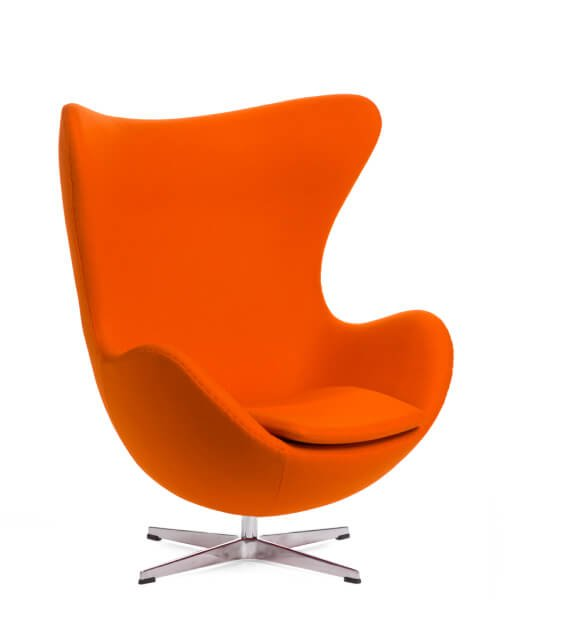•en

# What is Volume?

Volume is a measure of the three-dimensional space occupied by an object. In 3D visualization, it is often used to describe the size of an object or scene. For example, when looking at a building from above, the volume would be the height multiplied by the width multiplied by the length. Volume can also be used to describe the amount of data in a scene. For example, a scene with a lot of detail or a large number of objects would have a large volume.

Volume is an important concept in 3D visualization because it helps us understand the size and scale of objects and scenes. It can also be used to measure how much data is in a scene, which is important for understanding how complex a scene may be.Fill out the form
We will reply within 1 working day